# Thanks Consider the system y" 2by + y 0 with initial conditions y(0) 0, y (0)...

Thanks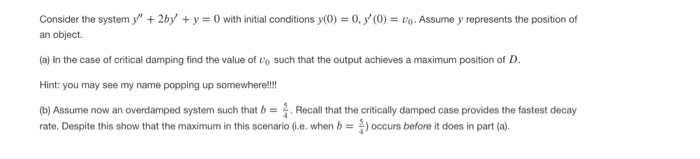Consider the system y" 2by + y 0 with initial conditions y(0) 0, y (0) = Uo. Assume y represents the position of an object (a) In the case of critical damping find the value of Uo such that the output achieves a maximum position of D Hint: you may see my name popping up somewhere!!! Recall that the critically damped case provides the fastest decay (b) Assume now an overdamped system such that b rate. Despite this show that the maximum in this scenario (i.e. when b) occurs before it does in part (a)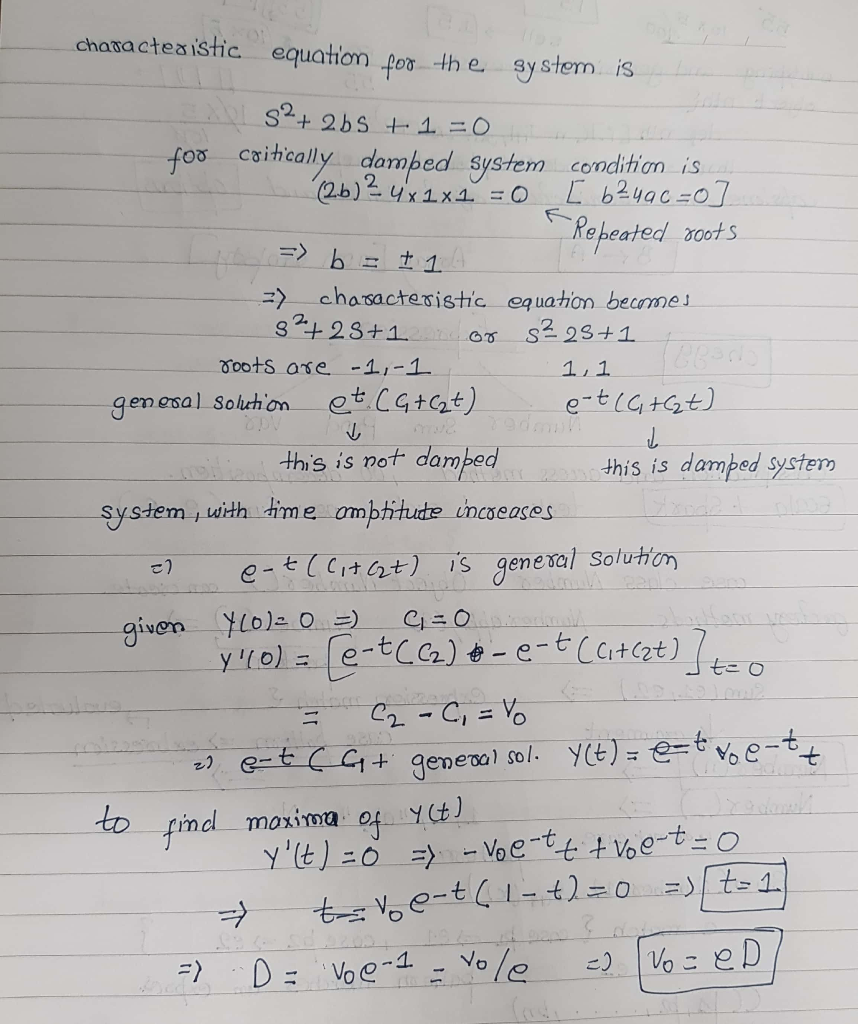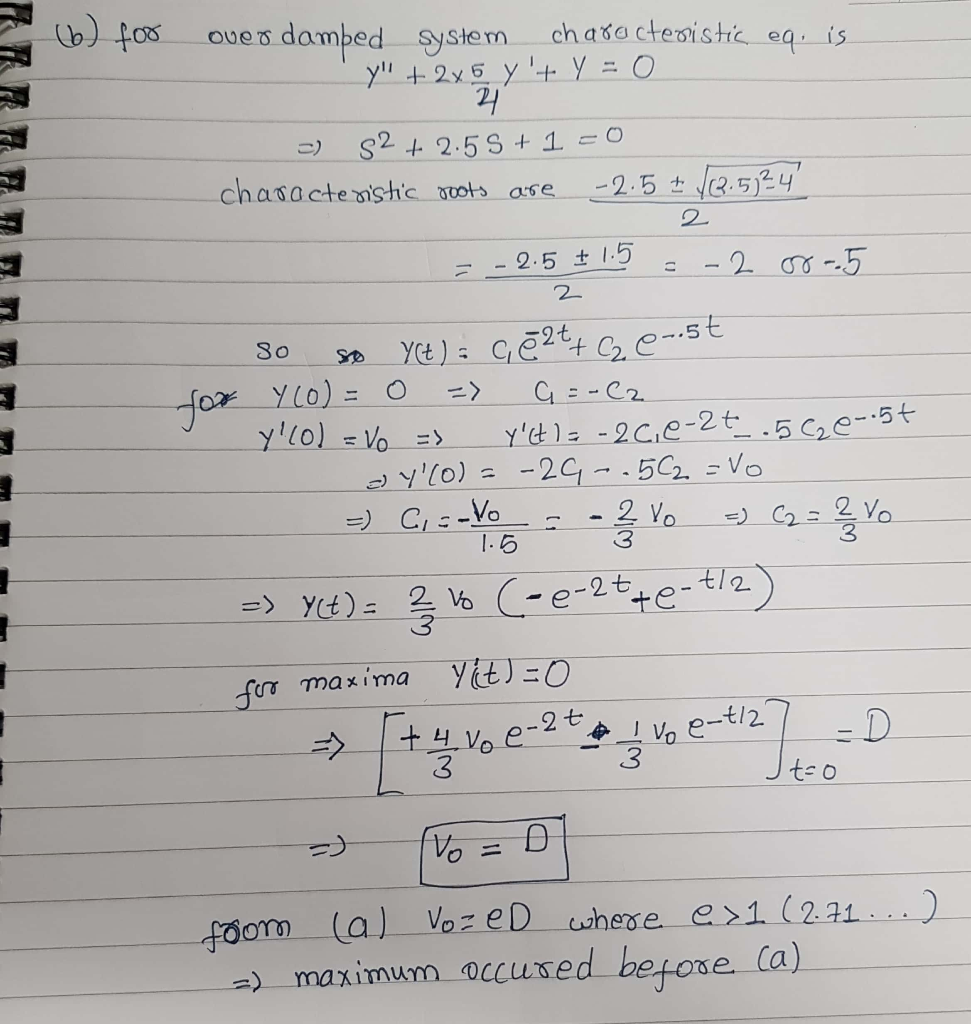##### Add Answer of: Thanks Consider the system y" 2by + y 0 with initial conditions y(0) 0, y (0)...
Similar Homework Help Questions
• ###  25 pts. A damped single degree of freedom system without applied forces is oscillating due to...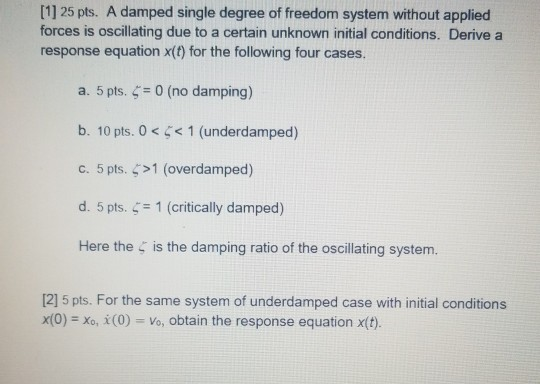solve for #2  25 pts. A damped single degree of freedom system without applied forces is oscillating due to a certain unknown initial conditions. Derive a response equation x(t) for the following four cases. a. 5 pts. 0 (no damping) b. 10 pts. 0<1 (underdamped) c. 5 pts. >1 (overdamped) d. 5 pts. ๕-1 (critically damped) Here the is the damping ratio of the oscillating system.  5 pts. For the same system of underdamped case with initial conditions...

• ### 1. The change of position of the center of mass of a rigid body in a mechanical system is being monitored. At time t 0, when the initial conditions of the system were x = 0.1 m and x -0m/s, a ste...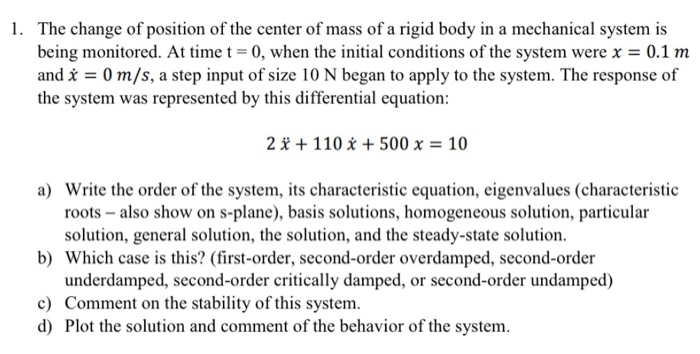1. The change of position of the center of mass of a rigid body in a mechanical system is being monitored. At time t 0, when the initial conditions of the system were x = 0.1 m and x -0m/s, a step input of size 10 N began to apply to the system. The response of the system was represented by this differential equation: 2r + 110x + 500 x = 10 a) Write the order of the system, its...

• ### 1) Answer the following questions for harmonic oscillator with the given parameters and initial c...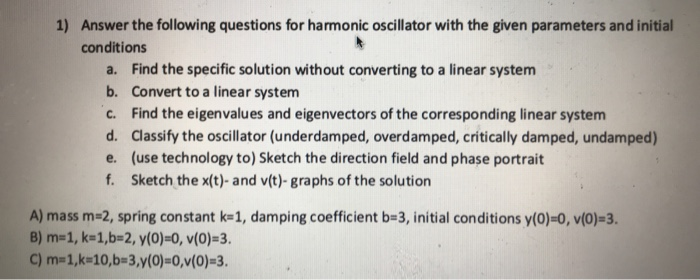1) Answer the following questions for harmonic oscillator with the given parameters and initial conditions Find the specific solution without converting to a linear system Convert to a linear system Find the eigenvalues and eigenvectors of the corresponding linear system Classify the oscillator (underdamped, overdamped, critically damped, undamped) (use technology to) Sketch the direction field and phase portrait Sketch the x(t)- and v(t)-graphs of the solution a. b. c. d. e. f. A) mass m-2, spring constant k 1, damping...

• ### 6. A mass of 2 kilogram is attached to a spring whose constant is 4 N/m, and the entire system is...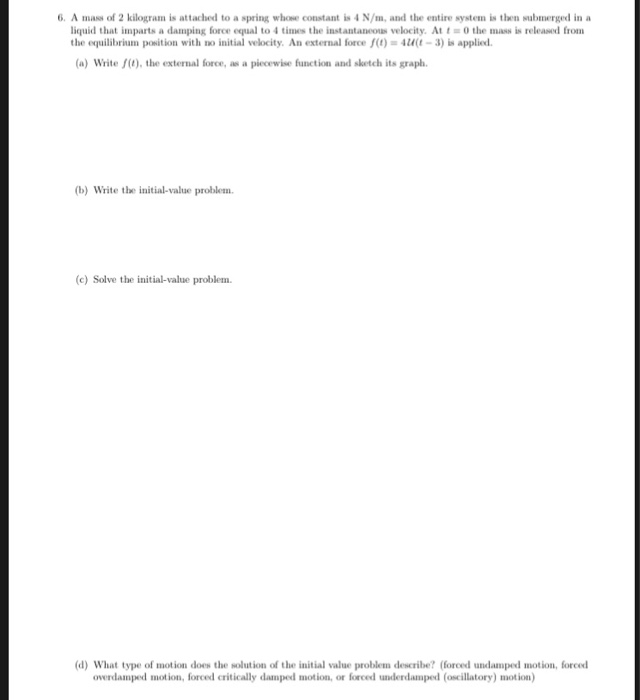6. A mass of 2 kilogram is attached to a spring whose constant is 4 N/m, and the entire system is then submerged in a liquid that inparts a damping force equal to 4 tines the instantansous velocity. At t = 0 the mass is released from the equilibrium position with no initial velocity. An external force t)4t-3) is applied. (a) Write (t), the external force, as a piecewise function and sketch its graph b) Write the initial-value problem (c)Solve...

• ### PROBLEMS 89 3-30. A system composed of a mass of S kg and an elastic member having a modulus of 4...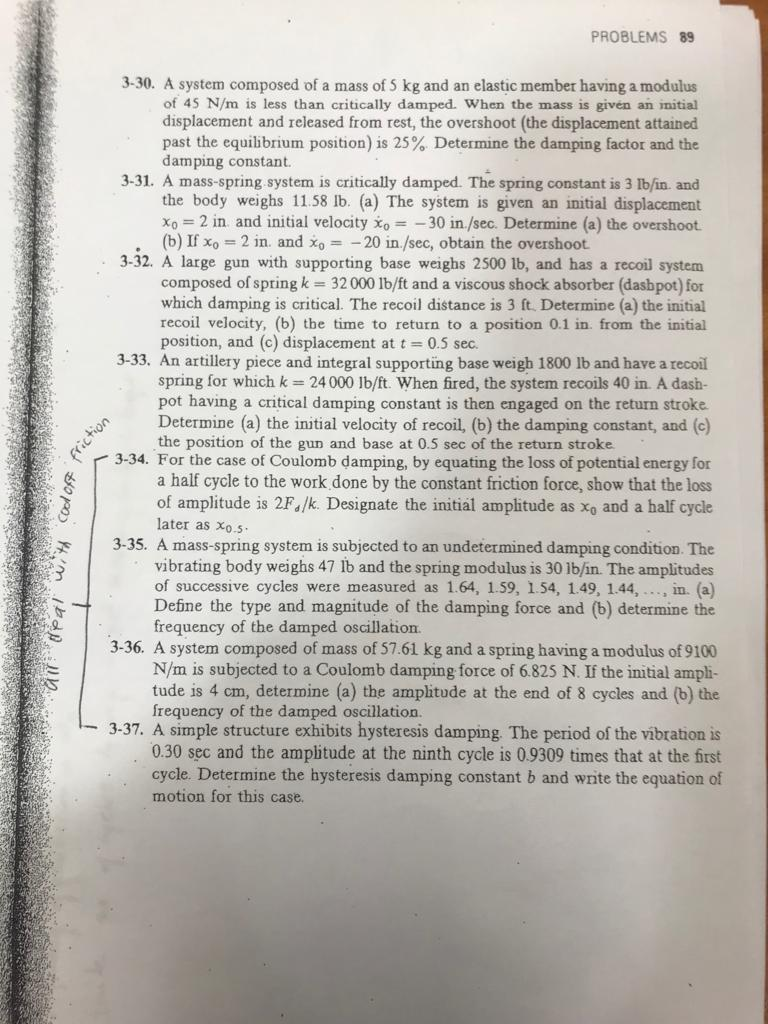Please answer 3-34 and 3-35. Please provide all steps so I can follow along. PROBLEMS 89 3-30. A system composed of a mass of S kg and an elastic member having a modulus of 45 N/m is less than critically damped. When the mass is givén an initial displacement and released from rest, the overshoot (the displacement attained past the equilibrium position) is 25% Determine the dam ping factor and the damping constant. 3-31. A mass-spring system is critically damped....

• ### ® Consider a damped unforced mass-spring system with m 1, γ 2, and k 26. a) (2 points) Find if th...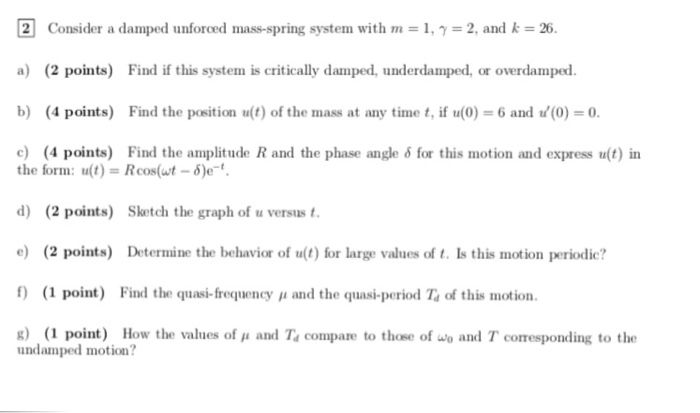solve d ,e , f, g ® Consider a damped unforced mass-spring system with m 1, γ 2, and k 26. a) (2 points) Find if this system is critically damped, underdamped, or overdamped. b) (4 points) Find the position u(t) of the mass at any time t if u(0)-6 and (0) 0. c) (4 points) Find the amplitude R and the phase angle δ for this motion and express u(t) in the form: u(t)-Rcos(wt -)e d) (2 points) Sketch...

• ### 5. A 2 kg mass is attached to a spring whose constant is 30 N/m, and the entire system is submerg...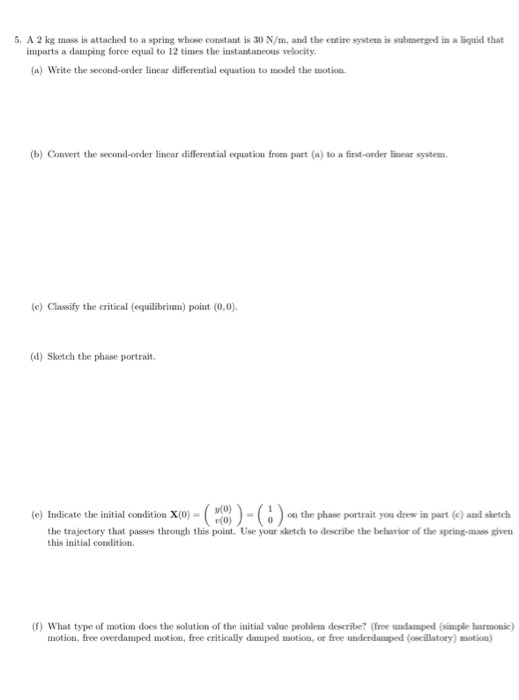5. A 2 kg mass is attached to a spring whose constant is 30 N/m, and the entire system is submerged in a liquid that imparts a damping force equal to 12 times the instaataneous velocity (a) Write the second-order linear differential equation to umodel the motion (b) Convert the second-order linear differential equation from part (a) to a first-order linear system (c) Classify the critical (equilibrium) point (0.0) (d) Sketch the phase portrait (e) Indicate the initial condition x(0)-(...

• ### MATLAB HELP (a) Use the command dsolve to find general solutions to the differential equations below. i. y 00 + 3y = 0 i...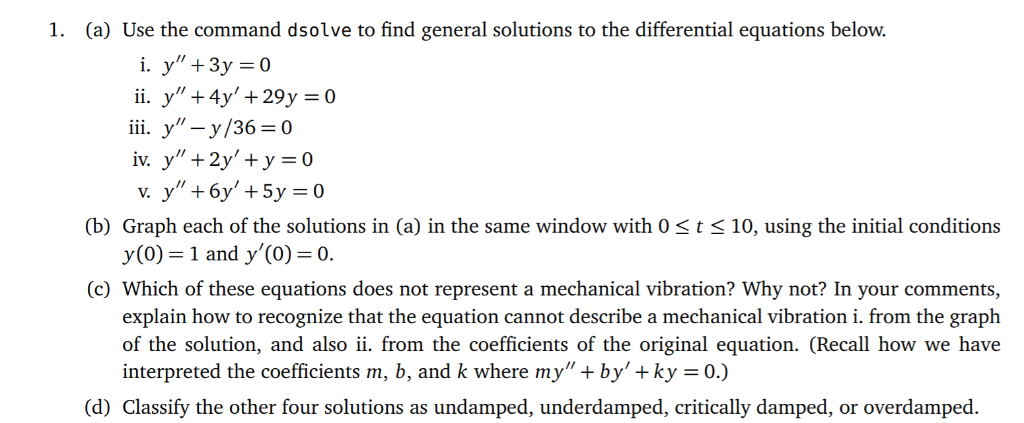MATLAB HELP (a) Use the command dsolve to find general solutions to the differential equations below. i. y 00 + 3y = 0 ii. y 00 + 4y 0 + 29y = 0 iii. y 00 − y/36 = 0 iv. y 00 + 2y 0 + y = 0 v. y 00 + 6y 0 + 5y = 0 (b) Graph each of the solutions in (a) in the same window with 0 ≤ t ≤ 10, using the...

• ### 6 (10) Spring Problems: (a) Find the displacement, y(t), (in arbitrary units) as a function of time for the mass in a mass-spring system described by the differential equatiorn Zy" 10y' +...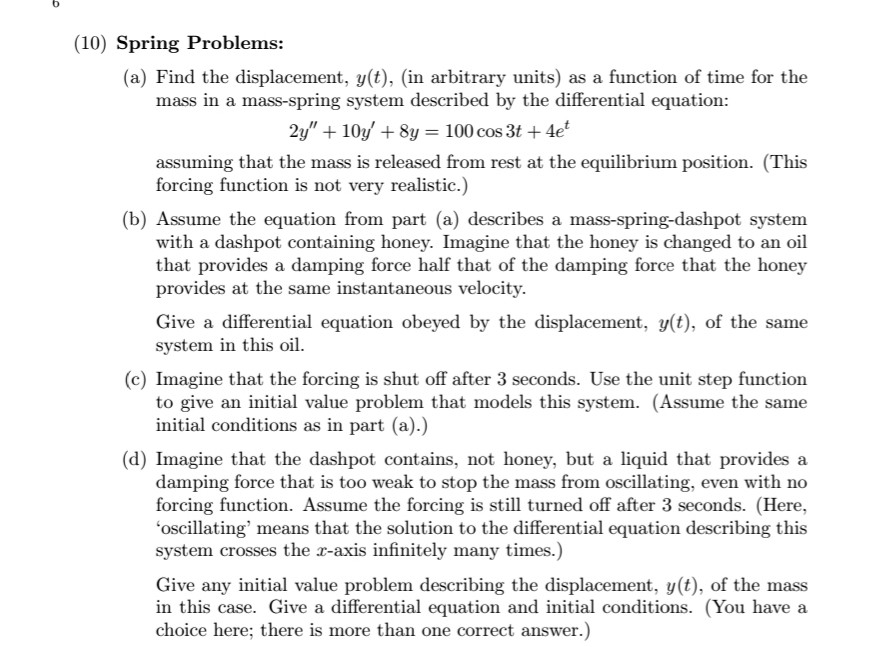6 (10) Spring Problems: (a) Find the displacement, y(t), (in arbitrary units) as a function of time for the mass in a mass-spring system described by the differential equatiorn Zy" 10y' + 8y = 100 cos 3t + 4et assuming that the mass is released from rest at the equilibrium position. (This forcing function is not very realistic.) (b) Assume the equation from part (a) describes a mass-spring-dashpot system with a dashpot containing honey. Imagine that the honey is changed...

• ### a-d please 6 (10) Spring Problems: (a) Find the displacement, y(t), (in arbitrary units) as a function of time for the mass in a mass-spring system described by the differential equatiorn Zy"...a-d please 6 (10) Spring Problems: (a) Find the displacement, y(t), (in arbitrary units) as a function of time for the mass in a mass-spring system described by the differential equatiorn Zy" 10y' + 8y = 100 cos 3t + 4et assuming that the mass is released from rest at the equilibrium position. (This forcing function is not very realistic.) (b) Assume the equation from part (a) describes a mass-spring-dashpot system with a dashpot containing honey. Imagine that the honey...

Need Online Homework Help?